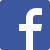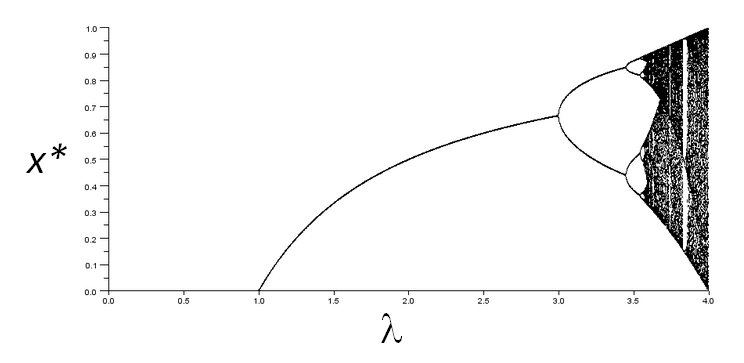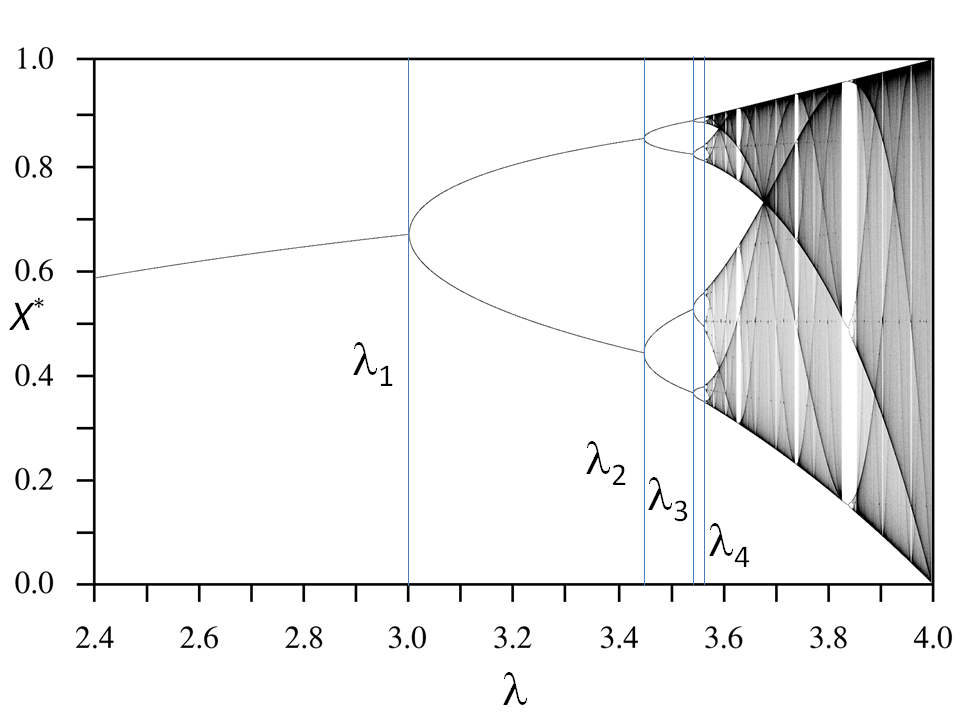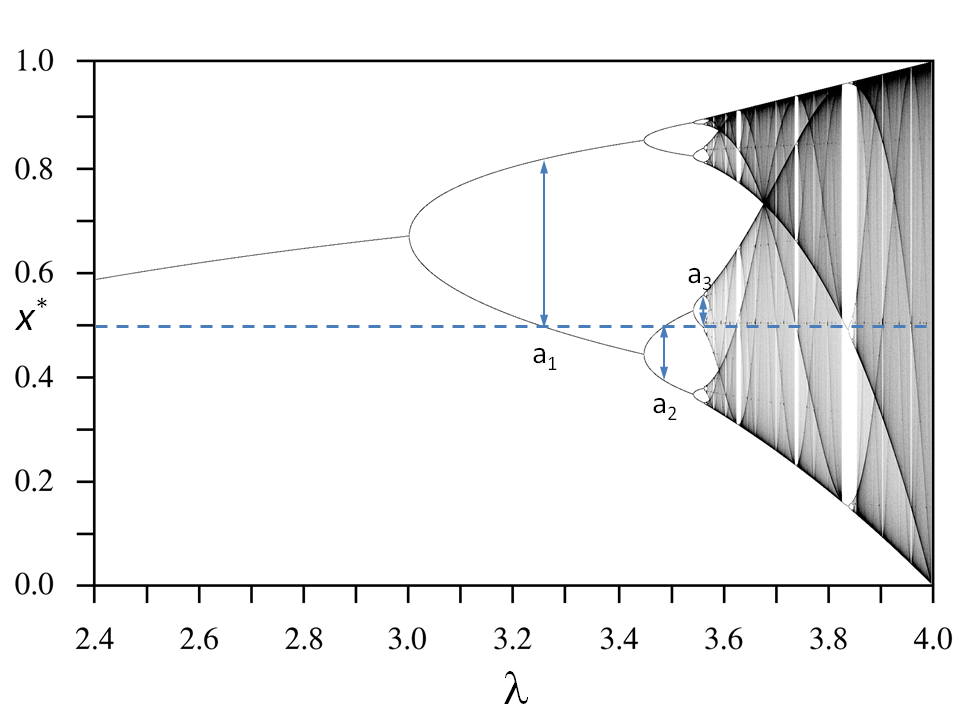Here is the latest from Julia Computing
BLG# A High Precision Calculation of Feigenbaum's Alpha Using Julia

27 Nov 2017 | Stuart Brorson, Dept of Mathematics, Northeastern University

The theory of dynamical systems has been an area of active research amongst physicists and mathematicians for several decades. Perhaps one of the most interesting objects of study in dynamical systems is the phenomenon of chaos.

Chaos is a phenomenon observed in some deterministic systems where the system’s time-dependent trajectory neither diverges, nor converges to a limit point, nor moves periodically. Rather, the system moves on an orbit which looks random.

A simple model system which evidences chaos is the so-called logistic map:

where $\lambda$ is a parameter allowed to vary over the domain $% $, and $x_n$ is the dynamical variable which varies over the domain $% $.

The idea is to choose a value of $\lambda$, then start with an arbitrary $x_0$, and insert it into the equation to get a new value, $x_1$. Then insert $x_1$ into the equation to get an update, $x_2$.

Repeat this process for large numbers of iterations and for many values of $\lambda$ the value $x_n$ converges to a value (or set of values). This value is called the “fixed point” of the iteration, typically written $x^\ast$. The value of $x^\ast$ depends upon $\lambda$.

A plot of the fixed point $x^\ast$ vs. $\lambda$ is shown in Figure 1 below. What’s interesting are different types of behavior obtained for different values of $\lambda$.

For $% $, $x$ converges to a single value. But starting at $\lambda = 3$, a new behavior emerges: instead of converging to one value, the variable $x$ hops from one value to a second one, then back.

For example, when $\lambda = 3.2$, $x$ hops between approximately $0.7995$ and $0.5130$. This is called a “period two” orbit, since $x$ visits two values, alternating with each iteration. Moreover, increasing $\lambda$ leads to a situation where $x$ visits 4 values, then 8 then 16, and eventually at a particular value of lambda, the period becomes infinity, meaning that $x$ has no periodic orbit. In this case, $x$ wanders around the unit interval quasi-randomly – the behavior we call chaos.Figure 1: The bifurcation diagram for the logistic map. (From Wikipedia.)

This transition from the period-one orbit to chaos is called the “period-doubling” route to chaos. It attracted the attention of many physicists in the 1970s and 80s since it offered the promise of illustrating a mechanism by which we could understand the development of complicated chaotic phenomena such as turbulence in fluids. Indeed, several physical systems were identified which evidenced a period-doubling route to chaos, including Duffing’s oscillator, a dripping faucet, and some simple electronic circuits. Unfortunately, the larger ambition of finally getting a grasp on turbulence by studying the logistic equation did not pan out. Nonetheless, some very interesting mathematics was discovered in the process.

In the late 1970s, Mitchell Feigenbaum, a mathematician at Los Alamos research laboratory, was playing around with the logistic equation using a hand calculator. He noticed some interesting numbers characterize the period doubling transition to chaos in the logistic map. He observed the following:

• Examine the length of each interval in $\lambda$ as the period doubles from $2 \rightarrow 4 \rightarrow 8 \rightarrow 16 \rightarrow 32$ etc. Feigenbaum found that the ratio of succeeding interval lengths converged to a value which he called $\delta$. The process is shown pictorially in Figure 2. Feigenbaum defined $\delta$ as $\delta = \lim_{n \to \infty } \frac{\lambda_n - \lambda_{n-1}}{\lambda_{n+1} - \lambda_n} \approx 4.6692$Figure 2: A close-up of the logistic map’s bifurcation diagram showing how $\delta$ is defined. (Figure adapted from Wikipedia.)

• Examine the width of the opening of each parabolic segment when it hits the value $x = 1/2$. (This value of $x$ is called the “superstable” point, and its value depends upon which map is under consideration. The value is 1/2 for the logistic map.) The ratio of successive widths also converges to a value. This is shown in Figure 3. Feigenbaum called this value $\alpha$. We have $\alpha = \lim_{n \to \infty } \frac{a_{n-1}}{a_n} \approx 2.502907$Figure 3: A close-up of the logistic map’s bifurcation diagram showing how $\alpha$ is defined. (Figure adapted from Wikipedia.)

Most importantly, Feigenbaum found that the value of these numbers was independent of the exact map used to generate period-doubling and chaos. Specifically, as long as the the map of the unit interval to itself,

is strictly concave and has a quadratic peak, then the map will evidence a period doubling transition to chaos characterized by numbers delta and alpha having exactly the values shown above.

For example, $\lambda x^2 (1 - x^2)$ and $\lambda \sin(x/\pi)$ behave the same as the logistic map. Of course, the exact location of the period doubling event occurs at different $\lambda$ values, but the ratios $\delta$ and $\alpha$ are the same. Therefore, the numbers $\delta$ and $\alpha$ are universal numerical constants on equal footing as the commonly known constants $\pi$, $e$, and $\gamma$.

This raises the question: How to calculate high-precision values for $\delta$ and $\alpha$?

I’ll save $\delta$ for a future post, and concentrate on $\alpha$ here. Using a renormalization group argument, Feigenbaum proposed that the stretching behavior of the logistic map around $x = 1/2$ was described by a universal function $g(z)$ which obeyed this equation in the limit:

and specifically, $\alpha$ could be found via $\alpha = 1/g(1)$. I won’t try to explain where this equation comes from here. Interested readers can read Feigenbaum’s summary paper .

My goal is to compute this function, and then use it to compute a high-precision value for $\alpha$.

## Previous work

I am aware of three earlier, high-precision computations of alpha. The first was performed by Australian mathematician Keith Briggs in his PhD thesis . He computed 576 decimal places (of which 344 were later found to be correct). The second was performed by UK mathematician David Broadhurst in 1999 , and is linked from the OEIS. He computed 1018 digits (after the decimal point). Finally, while I was preparing this post Professor Broadhurst pointed me to a paper by Andrea Molteni  who used a Chebyshev expansion instead of a Taylor’s expansion for $g(z)$ and got 10,000 digits.

## Julia

I became interested in chaos as an undergraduate, my curiosity including learning about the universal numbers $\delta$ and $\alpha$.

Decades later, when I became aware of Julia and its capabilities, I realized that it has some features which make it a good tool for computing these numbers. Julia’s important features include:

• Support for BigFloat. Prior computations used C or other low-level languages and manually linked to arbitrary-precision floating point libraries. This is complicated and error-prone. Julia makes it easy: Just use big() or BigFloat when declaring your high-precision numbers, and the BigFloat type will propagate through the rest of the calculation.

• Autodiff support. Like Briggs and Broadhurst, I employ the multidimensional Newton’s method to find the universal function $g(z)$ above. This involves computing a Jacobian. Computing a Jacobian by hand is also complicated and error-prone. Fortunately, over the last ten years or so, automatic differentiation techniques using dual numbers have become commonplace . Even better, Julia makes a number of autodiff packages available; they are gathered at the website http://www.juliadiff.org/. For my calculation I used the package ForwardDiff.jl.

With these features in mind, here is the method I used to compute alpha. It is the same used by Briggs and by Broadhurst, except that I implemented the computation in Julia.

• Expand $g(z)$ using an even power series to order $2N$: $g(z) = \sum_{n=1}^{\infty} a_n x^{2n}$

• Define a function $f(z)$ from the equation for $g(z)$. I will do root finding on $f(z)$ using Newton’s method on the interval $% $. We have, $f(z) = g(z) - \alpha g(g(z/\alpha)) = 0$

• Evaluate $f(z)$ at $N$ discrete points $z_i$, $i=1, ....N$. This gives $N$ equations in $N$ unknowns (the unknowns are the expansion coefficients $a_i$). The system of equations is $f(z_1; a_1, a_2, a_3 ...) = 0 \\ f(z_2; a_1, a_2, a_3 ...) = 0 \\ f(z_3; a_1, a_2, a_3 ...) = 0 \\ \vdots$

• Then use Newton’s method to solve the system. I use gradient.jl (part of the ForwardDiff.jl package) to compute the gradient of $f(z)$ at each $z_i$, then assemble the gradients into a Jacobian matrix. Newton’s method returns the expansion coefficients $a_i$.

• I wrap Newton’s method with an outer loop which calls it repeatedly. I start by asking for only, say, 10 digits. Newton’s method performs the solve then returns the expansion coefficients used to get the 10 digits. I then call Newton’s method again, but ask for more digits, say 30. I use the previously-found coefficients as the starting point for the new computation. This helps keep Newton’s method from wandering away from the desired solution as the number of requested digits increases. It is also useful when validating my result since I have a sequence of converging values which I can compare to each other.

My code to calculate alpha is available on GitHub for you to peruse, Here is the result of a typical run. As you see, I start by requesting a small number of digits, then walk up the requested precision. The variable $N$ reports the requested precision. Note that the number of converged digits is usually somewhat larger than the requested precision.

=========
N = 10
-2.5029078750941558550433538847562588590939864154825157840834005501784649816887308647883415497467233528729158301607769681388012068696297105559293578143978
==========
N = 30
-2.50290787509589282228390287321821578646680176860039691031775654917766795265509729744526592646450042740446507878591787900049867737721570507320536950555730233579295835513049755147443371058966655808267708238725531317972934168867025986314993674780442067259540137373182289118085931144660762909479796000659221064009292629086715493687279575196998009641864622893829398787716116103377437075875363832302242567069139487987577674934100040362548883506087905376040253
==========
N = 50
-2.502907875095892822283902873218215786381271376727149977336192057101332548200937314996958507672489476908625081364772906241928997687752969884387075775409826023146215641547577466532741243269601282751925295432306116410106657184918989460966694448022967464829388745475907553581492862863985138991456223981472184668490051357340519579612964363612398303916281396319853356754143270841575980410327246872085525908340194148771845711576975756396502129782335009689753534469725319971417668188501056069881195819569086079634680349686470334534259147981662223720090416466839684581834935585761392847958319659186046886869906843047200313111109896554722068418988625250918702774965150788866328601960325890564496371195820144217636314897987844658619824730317832913871839316087814207


If you compare each successive result to the next one, you will see that the sequence converges – the N=10 result contains 11 converged digits (after the decimal point), the N=30 result contains 36 converged digits, and so on.

Here is my best result so far, 1177 digits. This includes new digits at the end, beyond those computed by Broadhurst.

2.50290787509589282228390287321821578638127137672714997733619205677923546317959020670329964974643383412959523186999585472394218237778544517927286331499337257811216359487950374478126099738059867123971173732892766540440103066983138346000941393223644906578899512205843172507873377463087853424285351988587500042358246918740820428170090171482305182162161941319985606612938274264970984408447010080545496779367608881264464068851815527093240075425064971570470475419932831783645332562415378693957125097066387979492654623137674591890981311675243422111013091312783716095115834123084150371649970202246812196440812166865274580430262457825610671501385218216449532543349873487413352795815351016583605455763513276501810781194836945957485023739823545262563277947539726990201289151664579394201989202488033940516996865514944773965338769797412323540617819896112494095990353128997733611849847377946108428833293833903950900891408635152562680338141466927991331074334970514354520134464342647520016213846107299226419943327729189777690538025968518850841613864279936834741390166705544353112159412076097447476975360415562684762316863202036958955323302591969942848633937659618260681047820499176267330237410


## Verification

To check my results, I wrote a Julia script which accepts two files holding computed digits. The script then starts at the beginning of each file, and counts the number of matching characters.

stest = readstring("alpha_me.txt") #reading the test file
s = ""
count = 0
for i in length(min(stest,sref)) #iterating to count matches
if(stest[i] == sref[i])
count = count + 1
s = s * string(stest[i])
end
end
println("alpha_me.txt has $(length(stest)) digits.") #printing results println("alpha_oeis.txt has$(length(sref)) digits.")
println("Test file agrees with reference file to $count digits.") println("Digits of agreement: alpha =$s")


Here’s a run comparing one of my results against the digits reported by Broadhurst:

alpha_me.txt has 2441 digits.
alpha_oeis.txt has 1021 digits.
Test file agrees with reference file to 1020 digits.
Digits of agreement: alpha =
2.5029078750958928222839028732182157863812713767271499773361920567792354631795902067032996497464338341295952318699958547239421823777854451792728633149933725781121635948795037447812609973805986712397117373289276654044010306698313834600094139322364490657889951220584317250787337746308785342428535198858750004235824691874082042817009017148230518216216194131998560661293827426497098440844701008054549677936760888126446406885181552709324007542506497157047047541993283178364533256241537869395712509706638797949265462313767459189098131167524342211101309131278371609511583412308415037164997020224681219644081216686527458043026245782561067150138521821644953254334987348741335279581535101658360545576351327650181078119483694595748502373982354526256327794753972699020128915166457939420198920248803394051699686551494477396533876979741232354061781989611249409599035312899773361184984737794610842883329383390395090089140863515256268033814146692799133107433497051435452013446434264752001621384610729922641994332772918977769053802596851


This result matches all of Broadhurst’s digits, giving good confidence that my results are correct. Then, as I compute higher and higher number of digits, I can compare successive files against one another to find out how many digits have converged. As mentioned above, my current best result (above) is 1177 digits.

## Conclusion

I am not yet the world’s record holder when it comes to computing $\alpha$ – that honor belongs to Andrea Molteni who computed 10000 digits.

However, his calculation was written in C and linked to MPFR and MPI, so undoubtedly took some time and effort to write and debug. By taking advantage of Julia’s high-level features, I was able to write my program inside of a few hours.

1. Holmes, P., Whitley, D. “On the attracting set for Duffing’s equation”, Physica D, 111–123 (1983)

2. A. D’Innocenzo and L. Renna, “Modeling leaky faucet dynamics”, Phys. Rev. E, 55, 6676 (1997).

3. Paul Lindsay, “Period Doubling and Chaotic Behavior in a Driven Anharmonic Oscillator”, Physical Review Letters, 47 1349 (1981).

4. Mitchell J. Feigenbaum, “Universal Behavior in Nonlinear Systems,” in Los Alamos Science, Summer 1980, pp. 4-27 (available online at http://permalink.lanl.gov/object/tr?what=info:lanl-repo/lareport/LA-UR-80-5007).

5. Keith Briggs, “Feigenbaum scaling in discrete dynamical systems” (PhD Thesis, University of Melbourne, 1997) (available online via http://keithbriggs.info/thesis.html.

6. David Broadhurst, private communication. His results are available online at http://www.plouffe.fr/simon/constants/feigenbaum.txt.

7. Andrea Molteni, “An Efficient Method for the Computation of the Feigenbaum Constants to High Precision”, https://arxiv.org/pdf/1602.02357.pdf. His results are available online at http://converge.to/feigenbaum/.

8. Philipp H. W. Hoffmann, “A Hitchhiker’s Guide to Automatic Differentiation”, https://arxiv.org/pdf/1411.0583.pdf.

This is a guest blog post, written and edited by Stuart Brorson, Dept of Mathematics, Northeastern University, and formatted by Julia Computing’s Rajshekar Behar

## Recent posts

CSV Reader Benchmarks: Julia Reads CSVs 10-20x Faster than Python and R
22 Jun 2020 | Deepak Suresh
JuliaTeam v2.0 Now Available
12 Jun 2020 | Julia Computing